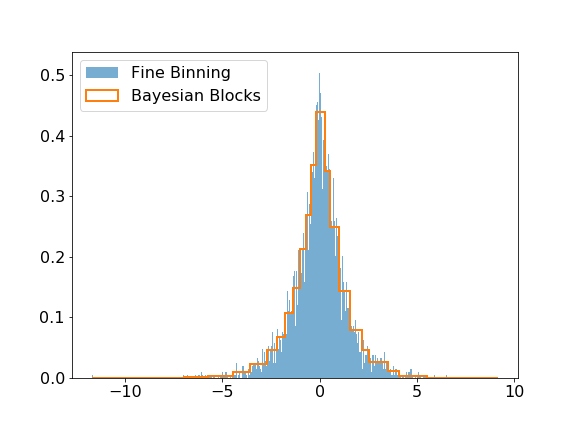Fetching contributors…Cannot retrieve contributors at this time
86 lines (62 sloc) 4.26 KB

# Scikit-HEP project `hepstats` package: statistics tools and utilities

## Installation

Install `hepstats` like any other Python package:

``````pip install hepstats
``````

or similar (use e.g. `virtualenv` if you wish).

## Getting Started

The `hepstats` module includes modeling and hypothesis tests submodules. This a quick user guide to each submodule. The binder examples are also a good way to get started.

### modeling

The modeling submodule includes the Bayesian Block algorithm that can be used to improve the binning of histograms. The visual improvement can be dramatic, and more importantly, this algorithm produces histograms that accurately represent the underlying distribution while being robust to statistical fluctuations. Here is a small example of the algorithm applied on Laplacian sampled data, compared to a histogram of this sample with a fine binning.

```>>> import numpy as np
>>> import matplotlib.pyplot as plt
>>> from hepstats.modeling import bayesian_blocks

>>> data = np.random.laplace(size=10000)
>>> blocks = bayesian_blocks(data)

>>> plt.hist(data, bins=1000, label='Fine Binning', density=True, alpha=0.6)
>>> plt.hist(data, bins=blocks, label='Bayesian Blocks', histtype='step', density=True, linewidth=2)
>>> plt.legend(loc=2)```### hypotests

This submodule provides tools to do hypothesis tests such as discovery test and computations of upper limits or confidence intervals. hepstats needs a fitting backend to perform computations such as zfit. Any fitting library can be used if their API is compatible with hepstats (see api checks).

We give here a simple example of a discovery test, using the zfit fitting package as backend, of a Gaussian signal with known mean and sigma over an exponential background.

```>>> import zfit
>>> from zfit.loss import ExtendedUnbinnedNLL
>>> from zfit.minimize import Minuit

>>> bounds = (0.1, 3.0)
>>> obs = zfit.Space('x', limits=bounds)

>>> bkg = np.random.exponential(0.5, 300)
>>> peak = np.random.normal(1.2, 0.1, 25)
>>> data = np.concatenate((bkg, peak))
>>> data = data[(data > bounds) & (data < bounds)]
>>> N = data.size
>>> data = zfit.Data.from_numpy(obs=obs, array=data)

>>> lambda_ = zfit.Parameter("lambda", -2.0, -4.0, -1.0)
>>> Nsig = zfit.Parameter("Ns", 20., -20., N)
>>> Nbkg = zfit.Parameter("Nbkg", N, 0., N*1.1)
>>> signal = Nsig * zfit.pdf.Gauss(obs=obs, mu=1.2, sigma=0.1)
>>> background = Nbkg * zfit.pdf.Exponential(obs=obs, lambda_=lambda_)
>>> loss = ExtendedUnbinnedNLL(model=signal + background, data=data)

>>> from hepstats.hypotests.calculators import AsymptoticCalculator
>>> from hepstats.hypotests import Discovery
>>> from hepstats.hypotests.parameters import POI

>>> calculator = AsymptoticCalculator(loss, Minuit())
>>> poinull = POI(Nsig, 0)
>>> discovery_test = Discovery(calculator, [poinull])
>>> discovery_test.result()

p_value for the Null hypothesis = 0.0007571045424956679
Significance (in units of sigma) = 3.1719464825102244```

The discovery test prints out the p-value and the significance of the null hypothesis to be rejected.

You can’t perform that action at this time.